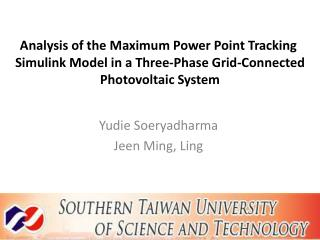# Yudie Soeryadharma Jeen Ming, Ling - PowerPoint PPT PresentationDownload PresentationDownload Presentation## Yudie Soeryadharma Jeen Ming, Ling

- - - - - - - - - - - - - - - - - - - - - - - - - - - E N D - - - - - - - - - - - - - - - - - - - - - - - - - - -
##### Presentation Transcript

1. Analysis of the Maximum Power Point Tracking Simulink Model in a Three-Phase Grid-Connected Photovoltaic System Yudie Soeryadharma Jeen Ming, Ling

2. ABSTRACT • Photovoltaic (PV) is one of the clean and free-pollution renewable energy, but it is an unreliable source because of the intermittent feature of weather. • Well integration of the MPPT technique with PV array model will ensure the system operates at its maximum power point at different weather conditions and solar irradiation. • This paper use the MATLAB Simulink to simulate the model.

3. INTRODUCTION • Recently, the needs of renewable energy resources increase due to the fuel energy crisis and the global warming issue. Solar energy is one of the most important renewable energy • Solar energy using photovoltaic (PV) has several advantages, e.g., no noise and free pollution. • Twooperational problems, the efficiency is very low especially under low irradiation conditions and in the intermittent weather condition the electric power changes continuously.

4. What is MPPT and why it is needed? (Maximum power point tracking) It is needed a controller (MPPT) to achieve the highest efficiency and provide a stable power under the intermittent weather condition • Why IC technique ? It shows good performance under the intermittent of solar irradiance The simulation result will show how good is the performance of this IC technique.

5. PV Model • A solar cell basically is a p-n semiconductor junction. When exposed to light, a dc current is generated.

6. PV Characteristic

7. IC MPPT Technique • The incremental conductance method is developed under the fact of slope of the PV array power curve is zero at the MPP IC MPPT Algorithm

8. Block Diagram of the Proposed PV System

9. SIMULATION SYSTEM

11. Simulation under constant solar irradiance • The maximum standard operating for constant solar irradiance is assumed to be 1000 W/m2 in the study. (A) The measured output of PV Module (B) The respond of IC MPPT tracking algorithm

12. Output from boost converter Under 1000 W/m2 of solar irradiance, The duty cycle for boost converter is a constant value 0.45 and the booster convert around 92kW of power with constant ouput voltage 500 V to the PV inverter.

13. Comparison of the measured output of PV module with output of boost converter The PV module provides 100kW and the boost converter convert 92kW of energy

14. Simulation under decreasing solar irradiance The figure shows the decreasing solar irradiance (1000 W/m2- 800 W/m2 -250 W/m2). (A) Decreasing of solar irradiance(B) Duty cycle of boost converter

15. (A) The measured output of PV Module (B) The respond of IC MPPT tracking algorithm

16. Output from boost converter The figure shows the power, voltage, current of boost converter. Under (1000 W/m2- 800 W/m2 -250 W/m2) of solar irradiance, the booster convert around 92kW - 80 kW - 25 kW of power. The booster maintains the output of voltage to be 500 V to the PV inverter.

17. Comparison of the measured output of PV module with output of boost converter The PV module provides 100kW (1000 W/m2) 80kW (800W/m2) 25kW (250 W/m2) and the boost converter convert 92kW, 74kW, 20 kW

18. Simulation under different solar irradiance The figure A shows the variations of solar irradiance (600 W/m2- 800 W/m2 - 400 W/m2 -600 W/m2) Figure B shows the duty cycle of boost converter responding to different solar irradiance. In fact, an extreme variation of solar irradiance occur rarely.

19. (A) The measured output of PV Module (B) The respond of IC MPPT tracking algorithm

20. Output from boost converter The figure shows the measurement output from boost converter. The figure shows the power, voltage, current of boost converter. Under (600 W/m2- 800 W/m2 - 400 W/m2 -600 W/m2) of solar irradiance, the booster maintains output voltage to be 500 V constantly.

21. Comparison of the measured output of PV module with output of boost converter The power efficiency is approximately 90%. The simulation result shows the MPPT works and respond fast and well even in any behavior of solar irradiance and also gain a high efficiency

22. PV module connected to grid The simulation is under 1000 W/m2 of solar irradiance. Output voltage of PV Inverter Output voltage of load

23. The figure shows the voltage after connected to the grid. to phase A-B in 25 kV voltage level. Output voltage of grid

24. Conclusion • In this paper, different solar radiations is used to investigate the performance of IC MPPT. • Simulation results show the IC MPPT technique in the PV system achieves the tracking of maximum power point with acceptable system performance and fast response. • For the future, the IC technique will be a reliable MPPT to apply in the real power system because of the intermittent of solar irradiance